Research papers and code for "Solomon Eyal Shimony":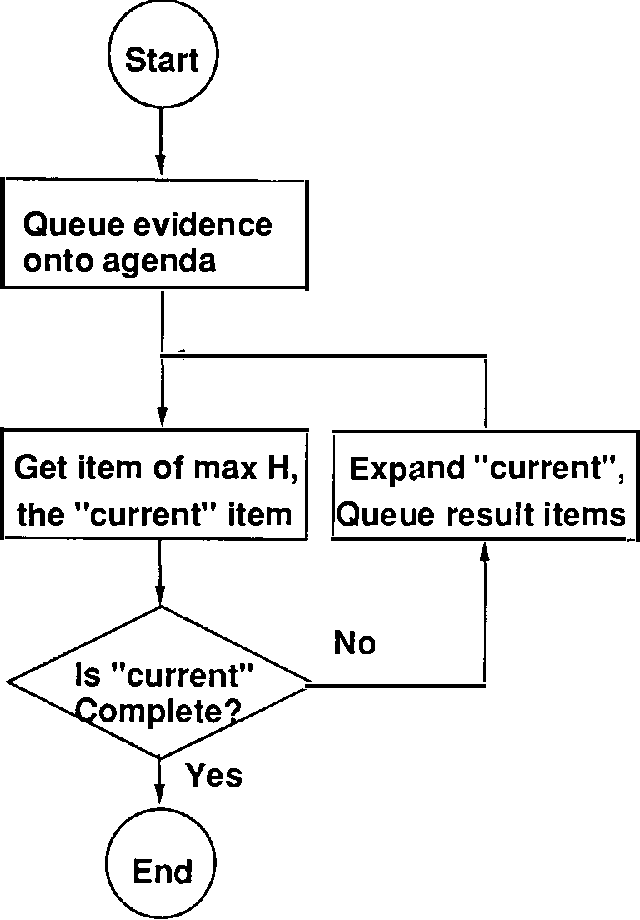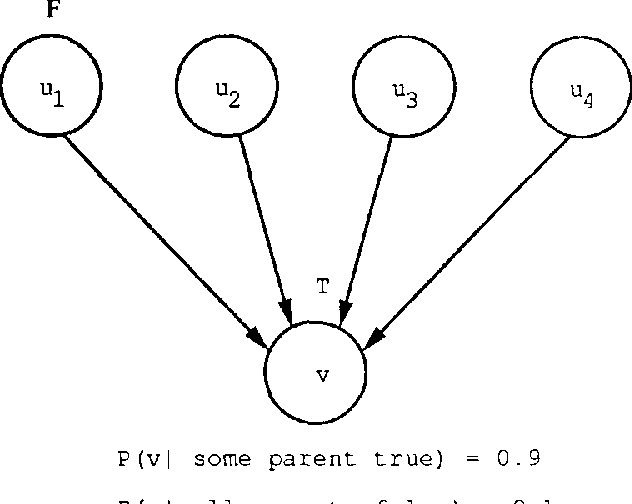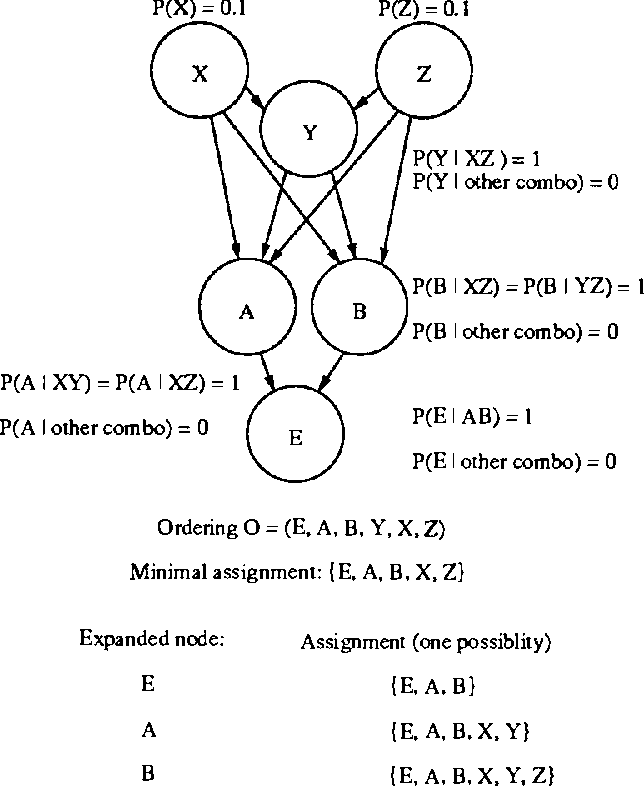Irrelevance-based partial MAPs are useful constructs for domain-independent explanation using belief networks. We look at two definitions for such partial MAPs, and prove important properties that are useful in designing algorithms for computing them effectively. We make use of these properties in modifying our standard MAP best-first algorithm, so as to handle irrelevance-based partial MAPs.

* Appears in Proceedings of the Seventh Conference on Uncertainty in Artificial Intelligence (UAI1991)
Click to Read Paper and Get Code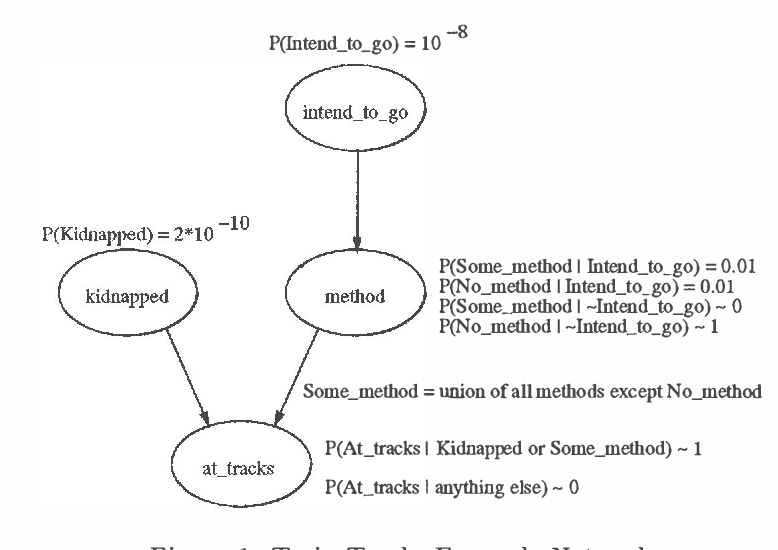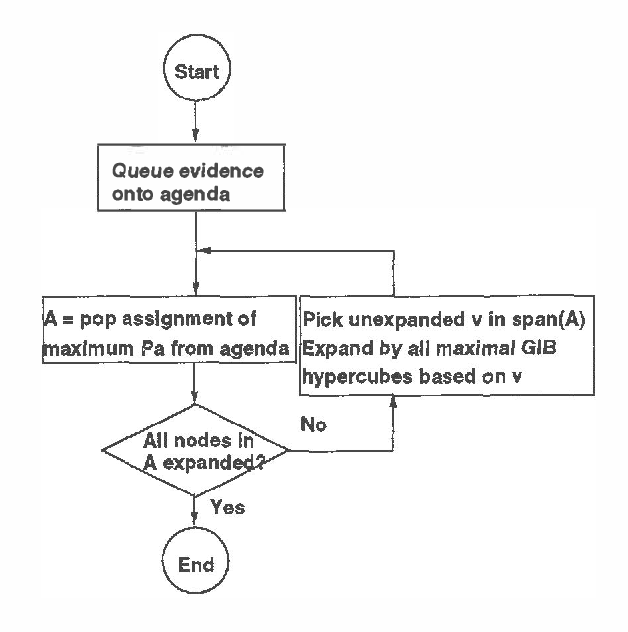Relevance-based explanation is a scheme in which partial assignments to Bayesian belief network variables are explanations (abductive conclusions). We allow variables to remain unassigned in explanations as long as they are irrelevant to the explanation, where irrelevance is defined in terms of statistical independence. When multiple-valued variables exist in the system, especially when subsets of values correspond to natural types of events, the over specification problem, alleviated by independence-based explanation, resurfaces. As a solution to that, as well as for addressing the question of explanation specificity, it is desirable to collapse such a subset of values into a single value on the fly. The equivalent method, which is adopted here, is to generalize the notion of assignments to allow disjunctive assignments. We proceed to define generalized independence based explanations as maximum posterior probability independence based generalized assignments (GIB-MAPs). GIB assignments are shown to have certain properties that ease the design of algorithms for computing GIB-MAPs. One such algorithm is discussed here, as well as suggestions for how other algorithms may be adapted to compute GIB-MAPs. GIB-MAP explanations still suffer from instability, a problem which may be addressed using ?approximate? conditional independence as a condition for irrelevance.

* Appears in Proceedings of the Ninth Conference on Uncertainty in Artificial Intelligence (UAI1993)
Click to Read Paper and Get Code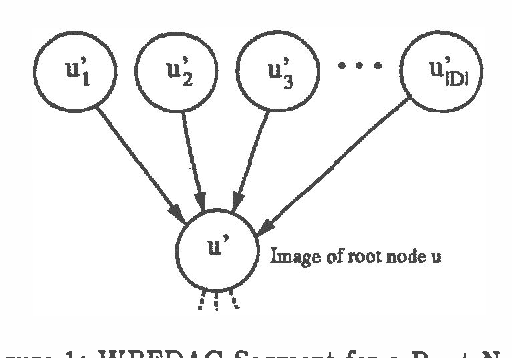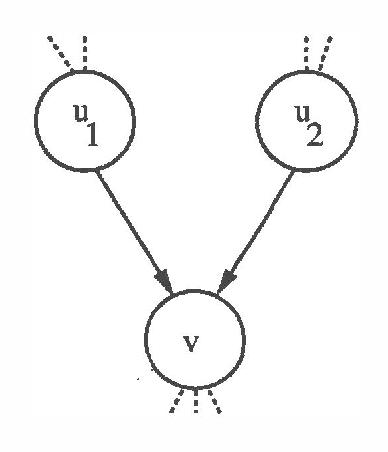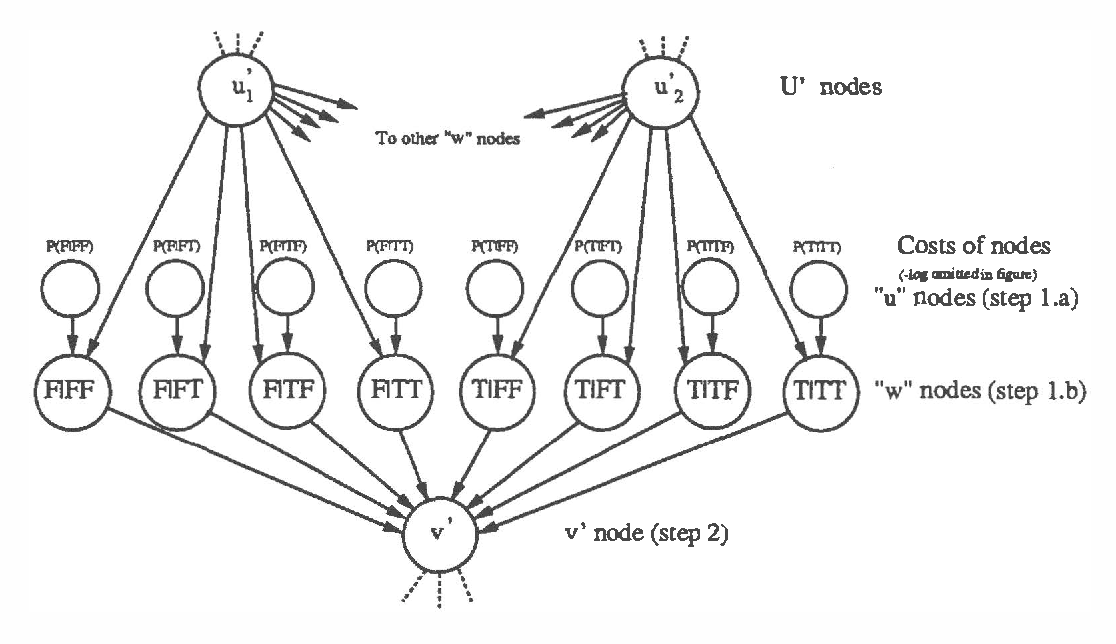We present a new algorithm for finding maximum a-posterior) (MAP) assignments of values to belief networks. The belief network is compiled into a network consisting only of nodes with boolean (i.e. only 0 or 1) conditional probabilities. The MAP assignment is then found using a best-first search on the resulting network. We argue that, as one would anticipate, the algorithm is exponential for the general case, but only linear in the size of the network for poly trees.

* Appears in Proceedings of the Sixth Conference on Uncertainty in Artificial Intelligence (UAI1990)
Click to Read Paper and Get Code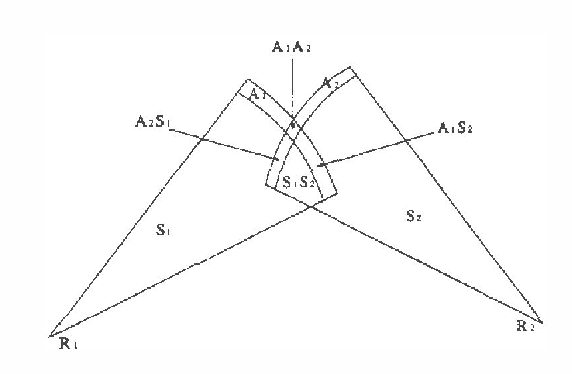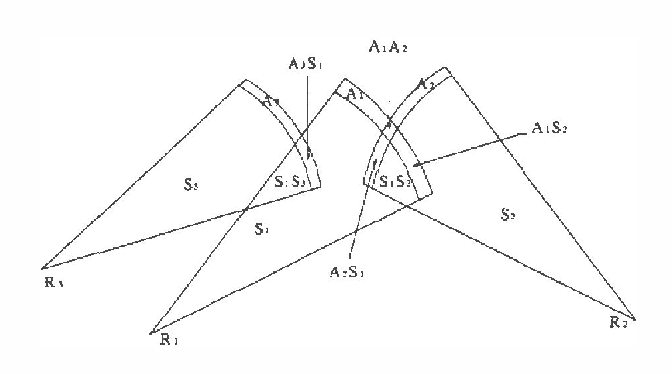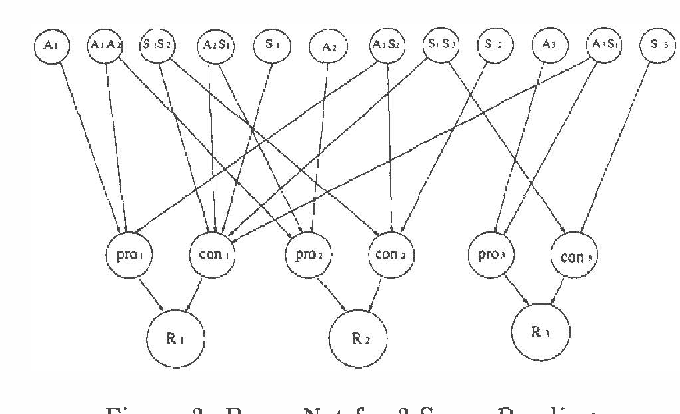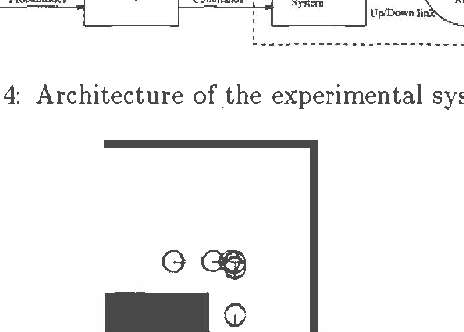Wide-angle sonar mapping of the environment by mobile robot is nontrivial due to several sources of uncertainty: dropouts due to "specular" reflections, obstacle location uncertainty due to the wide beam, and distance measurement error. Earlier papers address the latter problems, but dropouts remain a problem in many environments. We present an approach that lifts the overoptimistic independence assumption used in earlier work, and use Bayes nets to represent the dependencies between objects of the model. Objects of the model consist of readings, and of regions in which "quasi location invariance" of the (possible) obstacles exists, with respect to the readings. Simulation supports the method's feasibility. The model is readily extensible to allow for prior distributions, as well as other types of sensing operations.

* Appears in Proceedings of the Thirteenth Conference on Uncertainty in Artificial Intelligence (UAI1997)
Click to Read Paper and Get Code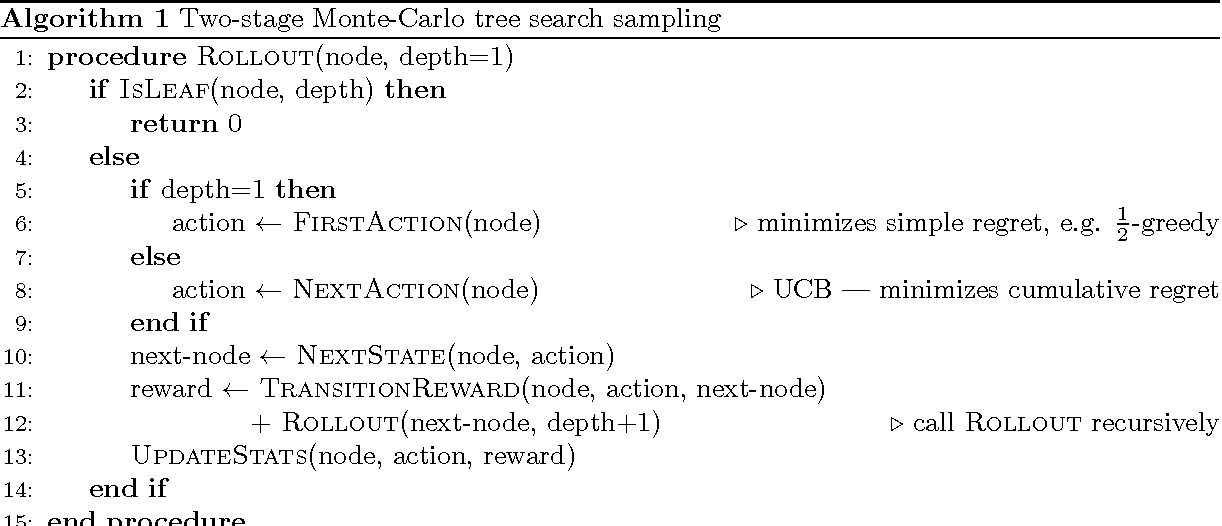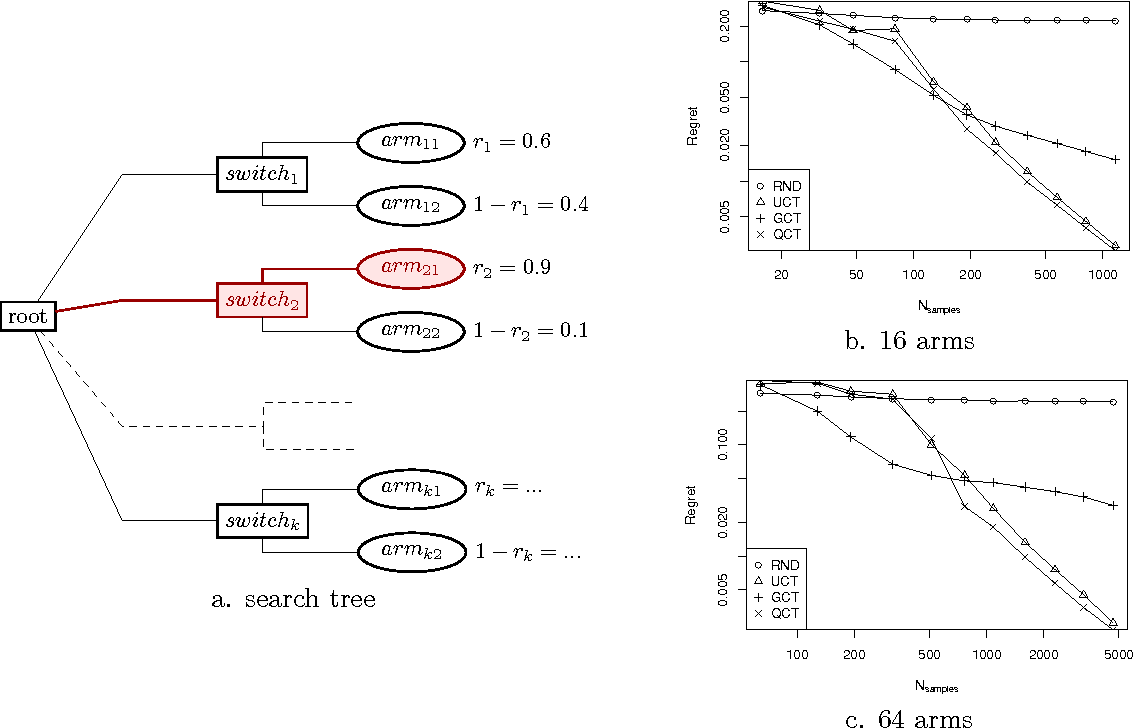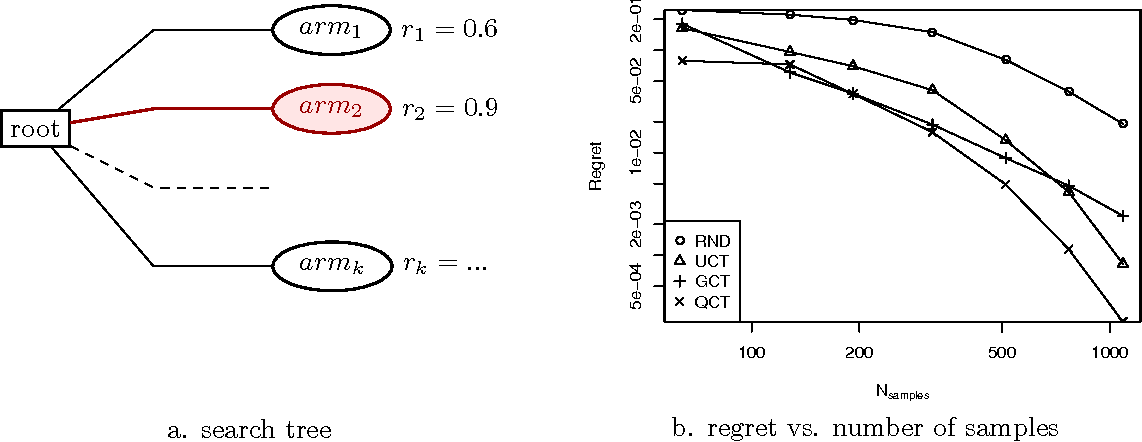UCT, a state-of-the art algorithm for Monte Carlo tree sampling (MCTS), is based on UCB, a sampling policy for the Multi-armed Bandit Problem (MAB) that minimizes the accumulated regret. However, MCTS differs from MAB in that only the final choice, rather than all arm pulls, brings a reward, that is, the simple regret, as opposite to the cumulative regret, must be minimized. This ongoing work aims at applying meta-reasoning techniques to MCTS, which is non-trivial. We begin by introducing policies for multi-armed bandits with lower simple regret than UCB, and an algorithm for MCTS which combines cumulative and simple regret minimization and outperforms UCT. We also develop a sampling scheme loosely based on a myopic version of perfect value of information. Finite-time and asymptotic analysis of the policies is provided, and the algorithms are compared empirically.

* Withdrawn: "MCTS Based on Simple Regret" (arXiv:1207.5589) is the final corrected version published in AAAI 2012 proceedings
Click to Read Paper and Get Code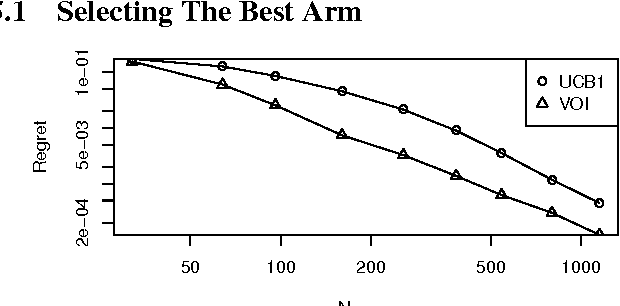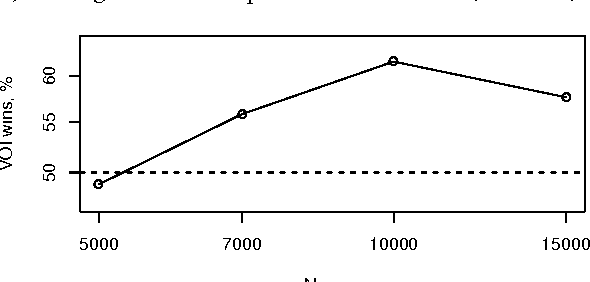UCT, a state-of-the art algorithm for Monte Carlo tree search (MCTS) in games and Markov decision processes, is based on UCB1, a sampling policy for the Multi-armed Bandit problem (MAB) that minimizes the cumulative regret. However, search differs from MAB in that in MCTS it is usually only the final "arm pull" (the actual move selection) that collects a reward, rather than all "arm pulls". In this paper, an MCTS sampling policy based on Value of Information (VOI) estimates of rollouts is suggested. Empirical evaluation of the policy and comparison to UCB1 and UCT is performed on random MAB instances as well as on Computer Go.

* 2 pages, ECAI 2012
Click to Read Paper and Get Code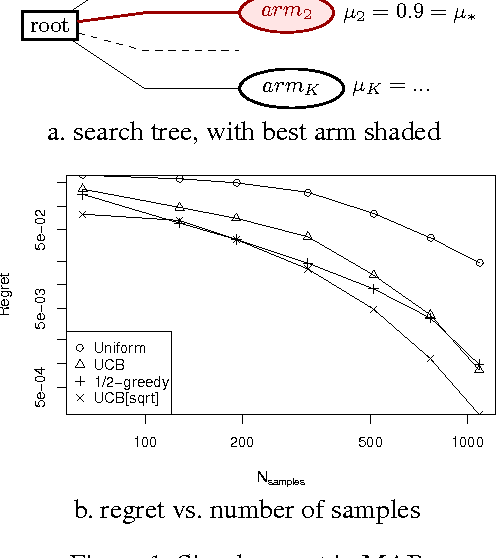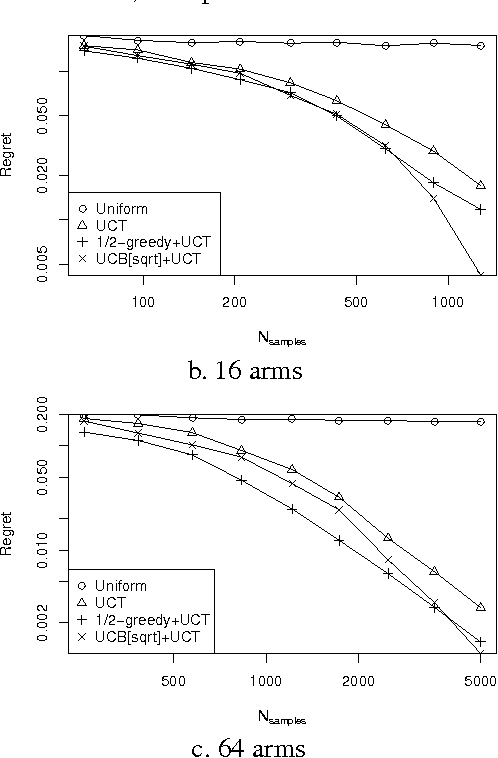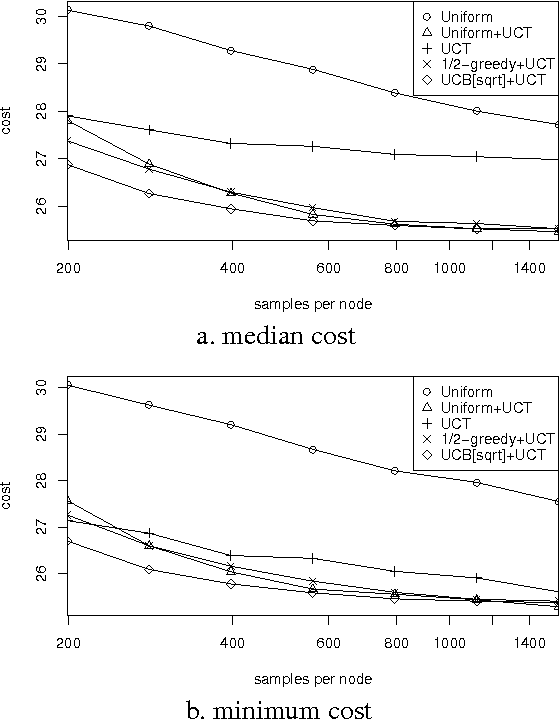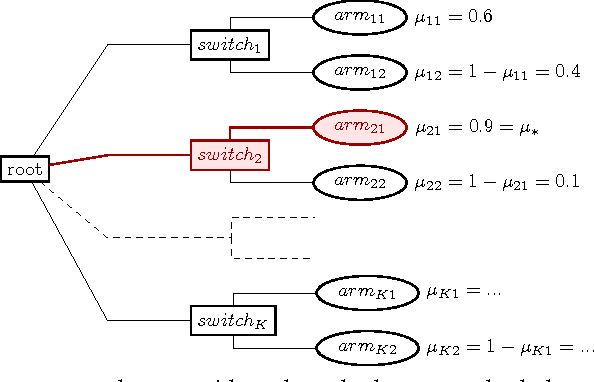UCT, a state-of-the art algorithm for Monte Carlo tree search (MCTS) in games and Markov decision processes, is based on UCB, a sampling policy for the Multi-armed Bandit problem (MAB) that minimizes the cumulative regret. However, search differs from MAB in that in MCTS it is usually only the final "arm pull" (the actual move selection) that collects a reward, rather than all "arm pulls". Therefore, it makes more sense to minimize the simple regret, as opposed to the cumulative regret. We begin by introducing policies for multi-armed bandits with lower finite-time and asymptotic simple regret than UCB, using it to develop a two-stage scheme (SR+CR) for MCTS which outperforms UCT empirically. Optimizing the sampling process is itself a metareasoning problem, a solution of which can use value of information (VOI) techniques. Although the theory of VOI for search exists, applying it to MCTS is non-trivial, as typical myopic assumptions fail. Lacking a complete working VOI theory for MCTS, we nevertheless propose a sampling scheme that is "aware" of VOI, achieving an algorithm that in empirical evaluation outperforms both UCT and the other proposed algorithms.

* AAAI 2012, 7 pages. arXiv admin note: text overlap with arXiv:1108.3711
Click to Read Paper and Get Code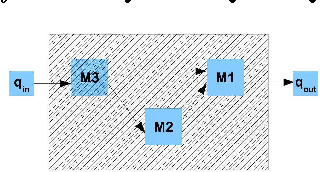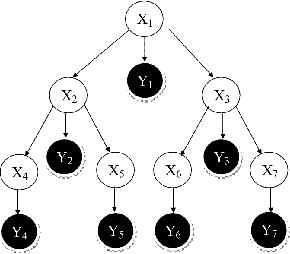Deciding what to sense is a crucial task, made harder by dependencies and by a nonadditive utility function. We develop approximation algorithms for selecting an optimal set of measurements, under a dependency structure modeled by a tree-shaped Bayesian network (BN). Our approach is a generalization of composing anytime algorithm represented by conditional performance profiles. This is done by relaxing the input monotonicity assumption, and extending the local compilation technique to more general classes of performance profiles (PPs). We apply the extended scheme to selecting a subset of measurements for choosing a maximum expectation variable in a binary valued BN, and for minimizing the worst variance in a Gaussian BN.

* Appears in Proceedings of the Twenty-Fourth Conference on Uncertainty in Artificial Intelligence (UAI2008)
Click to Read Paper and Get Code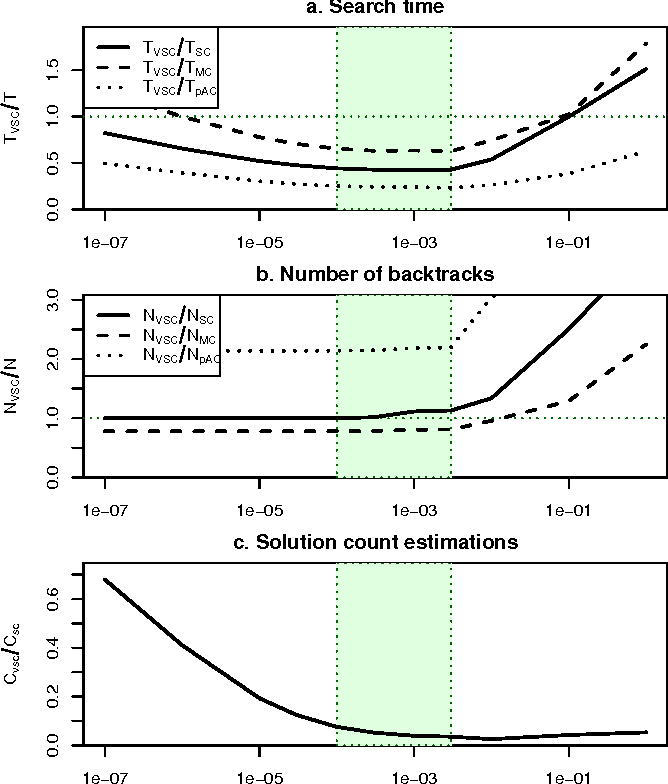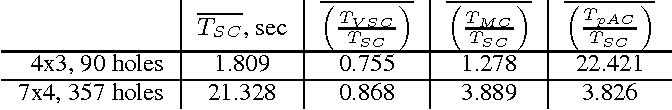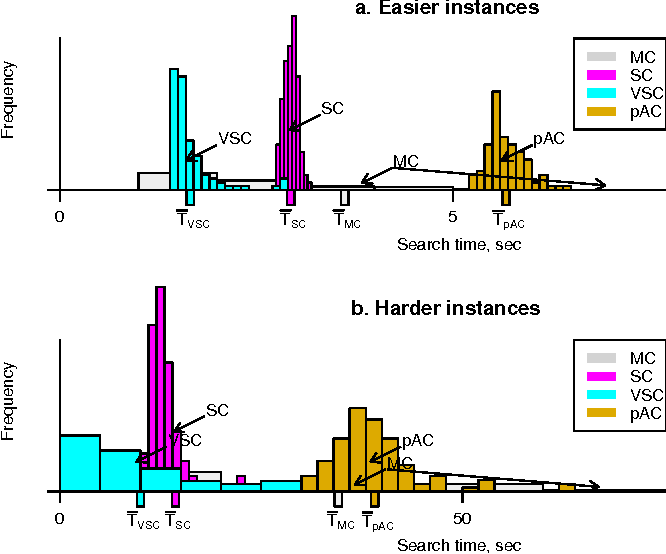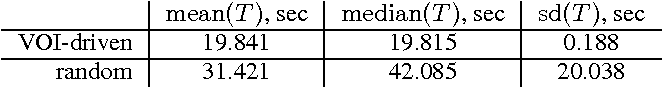Heuristics are crucial tools in decreasing search effort in varied fields of AI. In order to be effective, a heuristic must be efficient to compute, as well as provide useful information to the search algorithm. However, some well-known heuristics which do well in reducing backtracking are so heavy that the gain of deploying them in a search algorithm might be outweighed by their overhead. We propose a rational metareasoning approach to decide when to deploy heuristics, using CSP backtracking search as a case study. In particular, a value of information approach is taken to adaptive deployment of solution-count estimation heuristics for value ordering. Empirical results show that indeed the proposed mechanism successfully balances the tradeoff between decreasing backtracking and heuristic computational overhead, resulting in a significant overall search time reduction.

* IJCAI-2011
* 7 pages, 2 figures, to appear in IJCAI-2011, http://www.ijcai.org/
Click to Read Paper and Get Code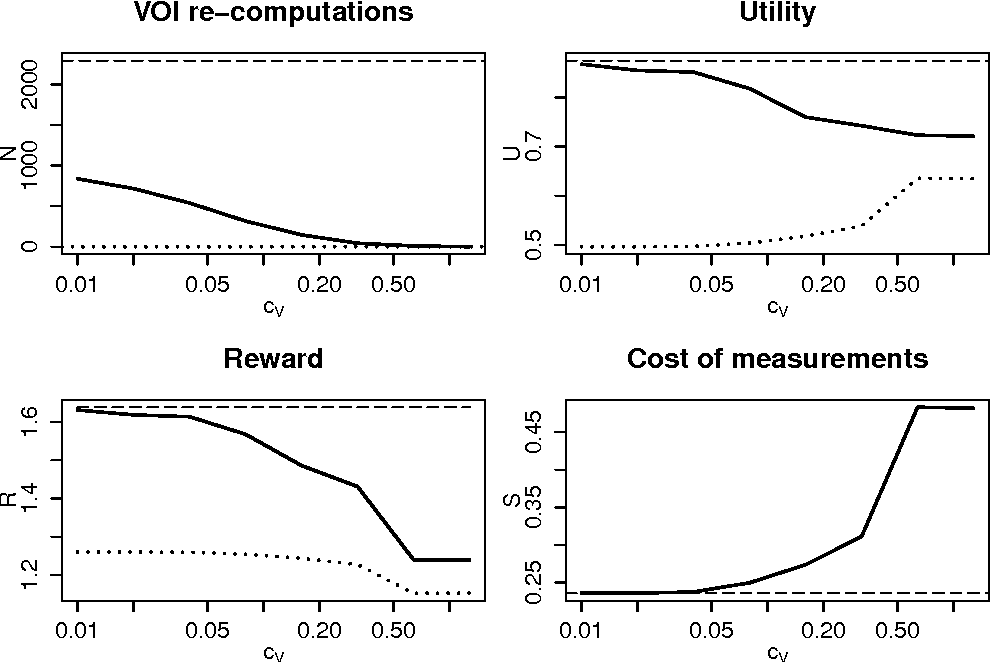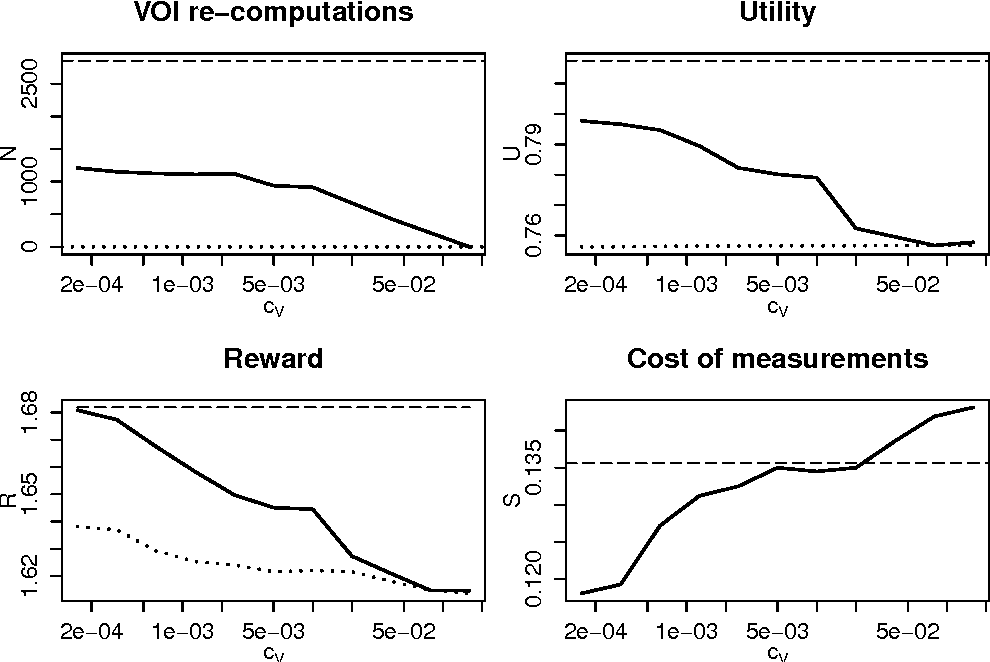Computing value of information (VOI) is a crucial task in various aspects of decision-making under uncertainty, such as in meta-reasoning for search; in selecting measurements to make, prior to choosing a course of action; and in managing the exploration vs. exploitation tradeoff. Since such applications typically require numerous VOI computations during a single run, it is essential that VOI be computed efficiently. We examine the issue of anytime estimation of VOI, as frequently it suffices to get a crude estimate of the VOI, thus saving considerable computational resources. As a case study, we examine VOI estimation in the measurement selection problem. Empirical evaluation of the proposed scheme in this domain shows that computational resources can indeed be significantly reduced, at little cost in expected rewards achieved in the overall decision problem.

* 7 pages, 2 figures, presented at URPDM2010; plots fixed
Click to Read Paper and Get Code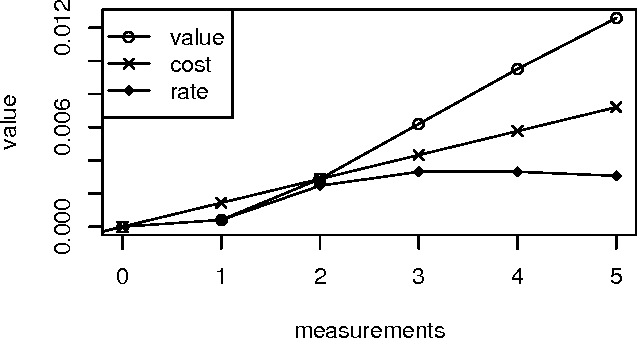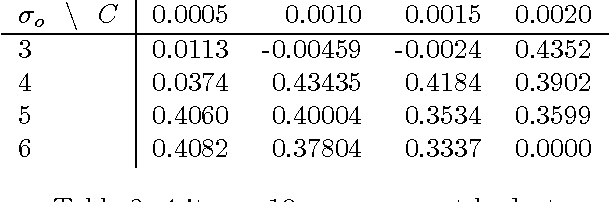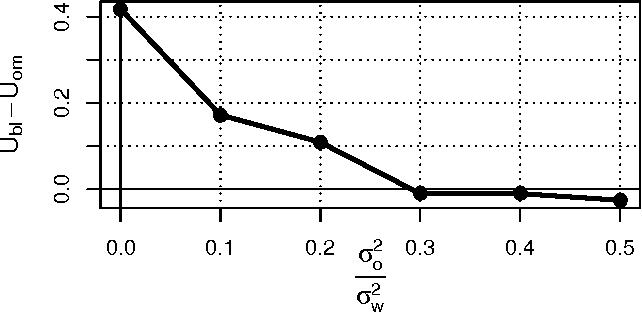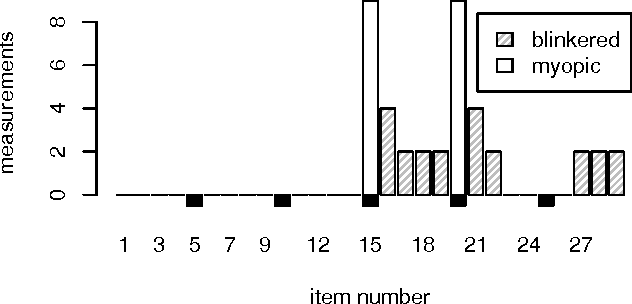We consider the following sequential decision problem. Given a set of items of unknown utility, we need to select one of as high a utility as possible (the selection problem''). Measurements (possibly noisy) of item values prior to selection are allowed, at a known cost. The goal is to optimize the overall sequential decision process of measurements and selection. Value of information (VOI) is a well-known scheme for selecting measurements, but the intractability of the problem typically leads to using myopic VOI estimates. In the selection problem, myopic VOI frequently badly underestimates the value of information, leading to inferior sensing plans. We relax the strict myopic assumption into a scheme we term semi-myopic, providing a spectrum of methods that can improve the performance of sensing plans. In particular, we propose the efficiently computable method of blinkered'' VOI, and examine theoretical bounds for special cases. Empirical evaluation of blinkered'' VOI in the selection problem with normally distributed item values shows that is performs much better than pure myopic VOI.

* 9 pages, 4 figures, presented at BISFAI 2009
Click to Read Paper and Get Code
Independence-based (IB) assignments to Bayesian belief networks were originally proposed as abductive explanations. IB assignments assign fewer variables in abductive explanations than do schemes assigning values to all evidentially supported variables. We use IB assignments to approximate marginal probabilities in Bayesian belief networks. Recent work in belief updating for Bayes networks attempts to approximate posterior probabilities by finding a small number of the highest probability complete (or perhaps evidentially supported) assignments. Under certain assumptions, the probability mass in the union of these assignments is sufficient to obtain a good approximation. Such methods are especially useful for highly-connected networks, where the maximum clique size or the cutset size make the standard algorithms intractable. Since IB assignments contain fewer assigned variables, the probability mass in each assignment is greater than in the respective complete assignment. Thus, fewer IB assignments are sufficient, and a good approximation can be obtained more efficiently. IB assignments can be used for efficiently approximating posterior node probabilities even in cases which do not obey the rather strict skewness assumptions used in previous research. Two algorithms for finding the high probability IB assignments are suggested: one by doing a best-first heuristic search, and another by special-purpose integer linear programming. Experimental results show that this approach is feasible for highly connected belief networks.

* Appears in Proceedings of the Tenth Conference on Uncertainty in Artificial Intelligence (UAI1994)
Click to Read Paper and Get Code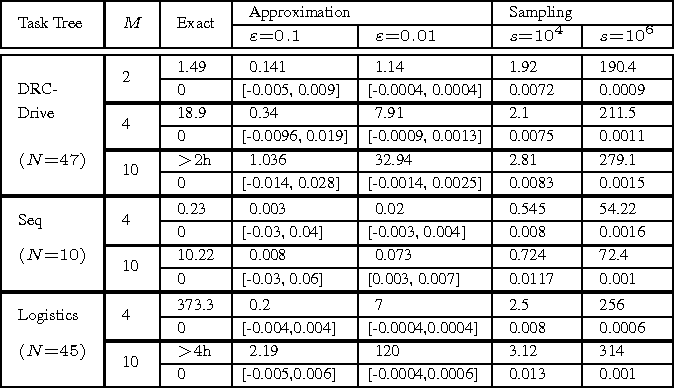Given a hierarchical plan (or schedule) with uncertain task times, we propose a deterministic polynomial (time and memory) algorithm for estimating the probability that its meets a deadline, or, alternately, that its {\em makespan} is less than a given duration. Approximation is needed as it is known that this problem is NP-hard even for sequential plans (just, a sum of random variables). In addition, we show two new complexity results: (1) Counting the number of events that do not cross deadline is \#P-hard; (2)~Computing the expected makespan of a hierarchical plan is NP-hard. For the proposed approximation algorithm, we establish formal approximation bounds and show that the time and memory complexities grow polynomially with the required accuracy, the number of nodes in the plan, and with the size of the support of the random variables that represent the durations of the primitive tasks. We examine these approximation bounds empirically and demonstrate, using task networks taken from the literature, how our scheme outperforms sampling techniques and exact computation in terms of accuracy and run-time. As the empirical data shows much better error bounds than guaranteed, we also suggest a method for tightening the bounds in some cases.

* A jornal version of an IJCAI-2015 paper: "Estimating the Probability of Meeting a Deadline in Hierarchical Plans"
Click to Read Paper and Get Code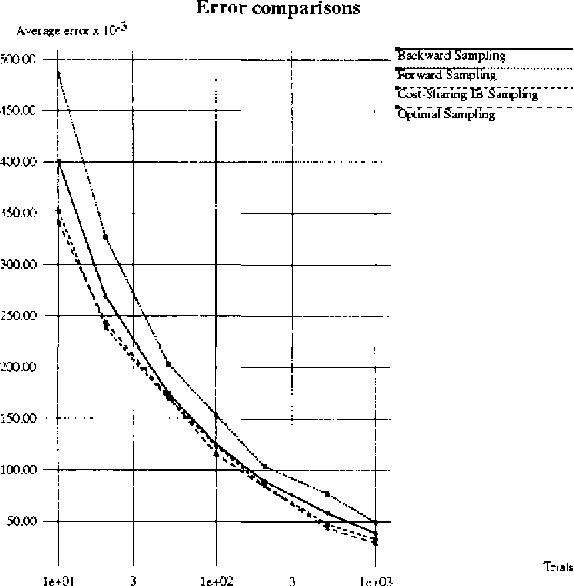Belief updating in Bayes nets, a well known computationally hard problem, has recently been approximated by several deterministic algorithms, and by various randomized approximation algorithms. Deterministic algorithms usually provide probability bounds, but have an exponential runtime. Some randomized schemes have a polynomial runtime, but provide only probability estimates. We present randomized algorithms that enumerate high-probability partial instantiations, resulting in probability bounds. Some of these algorithms are also sampling algorithms. Specifically, we introduce and evaluate a variant of backward sampling, both as a sampling algorithm and as a randomized enumeration algorithm. We also relax the implicit assumption used by both sampling and accumulation algorithms, that query nodes must be instantiated in all the samples.

* Appears in Proceedings of the Twelfth Conference on Uncertainty in Artificial Intelligence (UAI1996)
Click to Read Paper and Get Code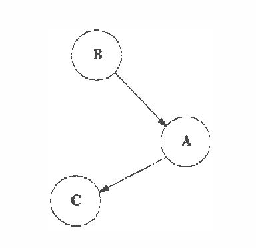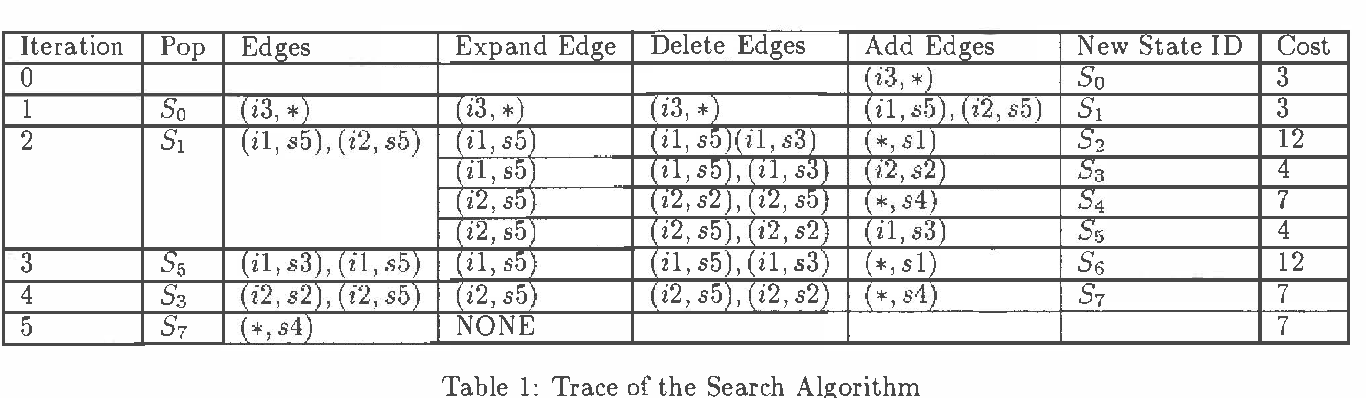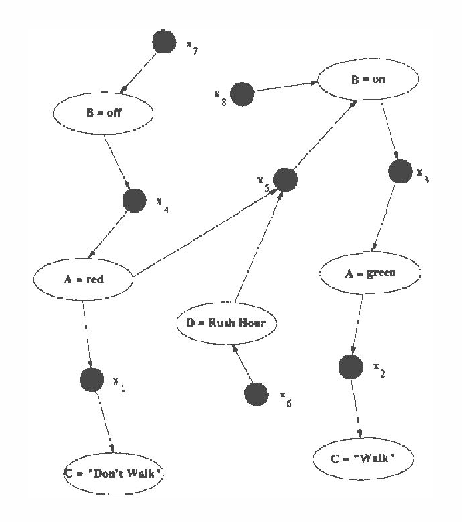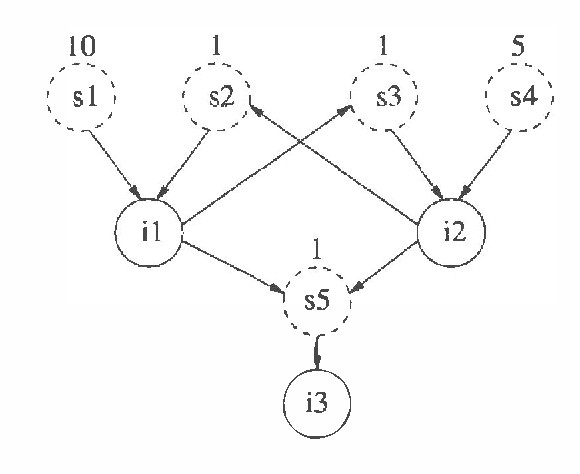Bayesian knowledge bases (BKBs) are a generalization of Bayes networks and weighted proof graphs (WAODAGs), that allow cycles in the causal graph. Reasoning in BKBs requires finding the most probable inferences consistent with the evidence. The cost-sharing heuristic for finding least-cost explanations in WAODAGs was presented and shown to be effective by Charniak and Husain. However, the cycles in BKBs would make the definition of cost-sharing cyclic as well, if applied directly to BKBs. By treating the defining equations of cost-sharing as a system of equations, one can properly define an admissible cost-sharing heuristic for BKBs. Empirical evaluation shows that cost-sharing improves performance significantly when applied to BKBs.

* Appears in Proceedings of the Thirteenth Conference on Uncertainty in Artificial Intelligence (UAI1997)
Click to Read Paper and Get Code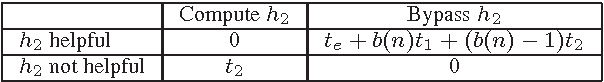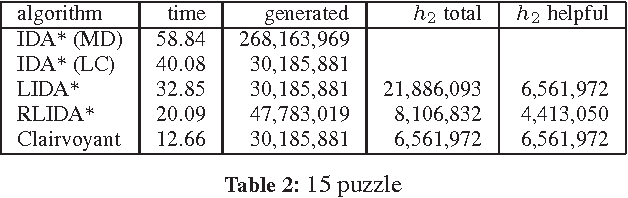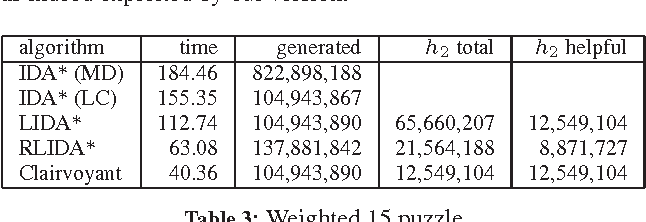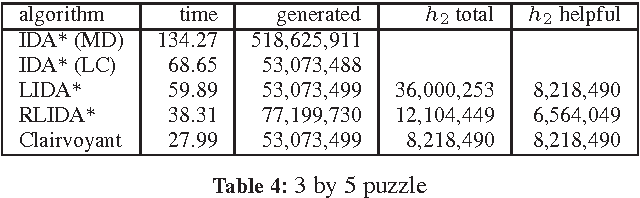Recent advances in metareasoning for search has shown its usefulness in improving numerous search algorithms. This paper applies rational metareasoning to IDA* when several admissible heuristics are available. The obvious basic approach of taking the maximum of the heuristics is improved upon by lazy evaluation of the heuristics, resulting in a variant known as Lazy IDA*. We introduce a rational version of lazy IDA* that decides whether to compute the more expensive heuristics or to bypass it, based on a myopic expected regret estimate. Empirical evaluation in several domains supports the theoretical results, and shows that rational lazy IDA* is a state-of-the-art heuristic combination method.

* 7 pages, 6 tables, 20 references
Click to Read Paper and Get Code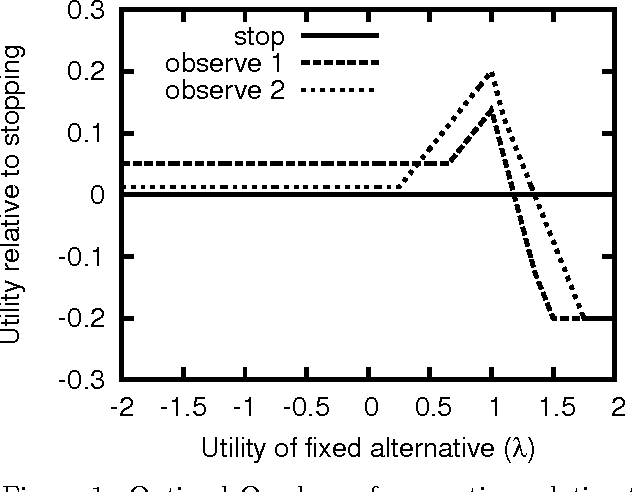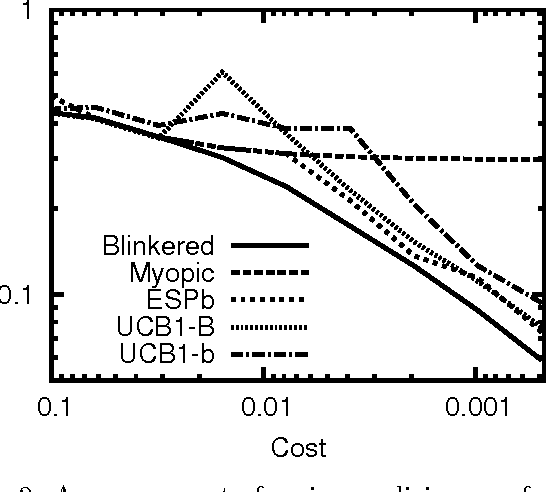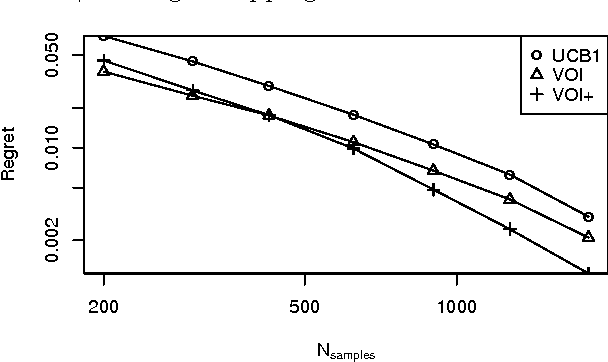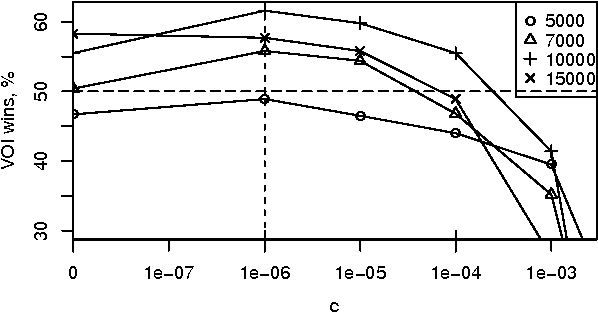Sequential decision problems are often approximately solvable by simulating possible future action sequences. Metalevel decision procedures have been developed for selecting which action sequences to simulate, based on estimating the expected improvement in decision quality that would result from any particular simulation; an example is the recent work on using bandit algorithms to control Monte Carlo tree search in the game of Go. In this paper we develop a theoretical basis for metalevel decisions in the statistical framework of Bayesian selection problems, arguing (as others have done) that this is more appropriate than the bandit framework. We derive a number of basic results applicable to Monte Carlo selection problems, including the first finite sampling bounds for optimal policies in certain cases; we also provide a simple counterexample to the intuitive conjecture that an optimal policy will necessarily reach a decision in all cases. We then derive heuristic approximations in both Bayesian and distribution-free settings and demonstrate their superiority to bandit-based heuristics in one-shot decision problems and in Go.

* Appears in Proceedings of the Twenty-Eighth Conference on Uncertainty in Artificial Intelligence (UAI2012)
Click to Read Paper and Get Code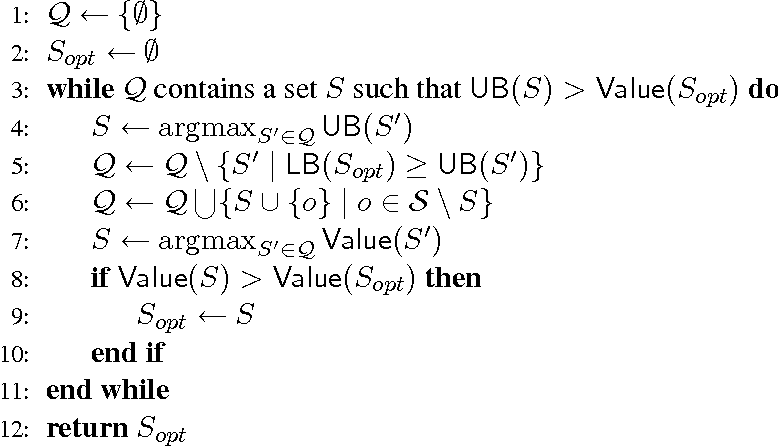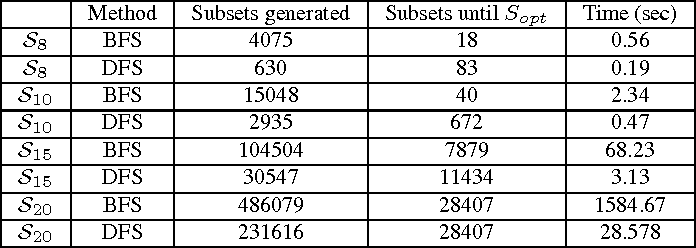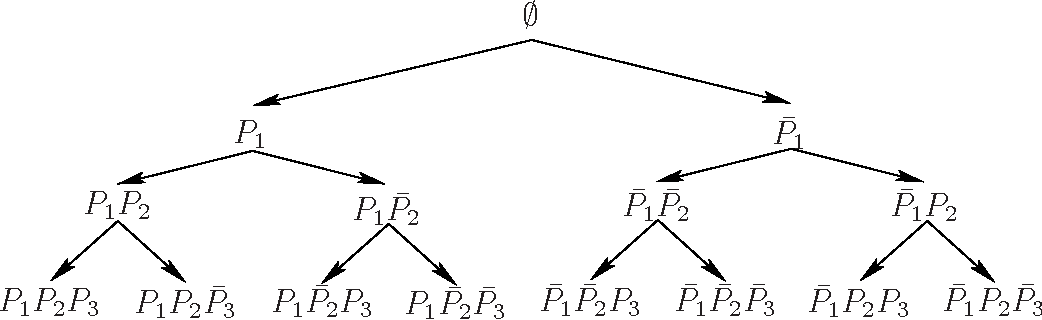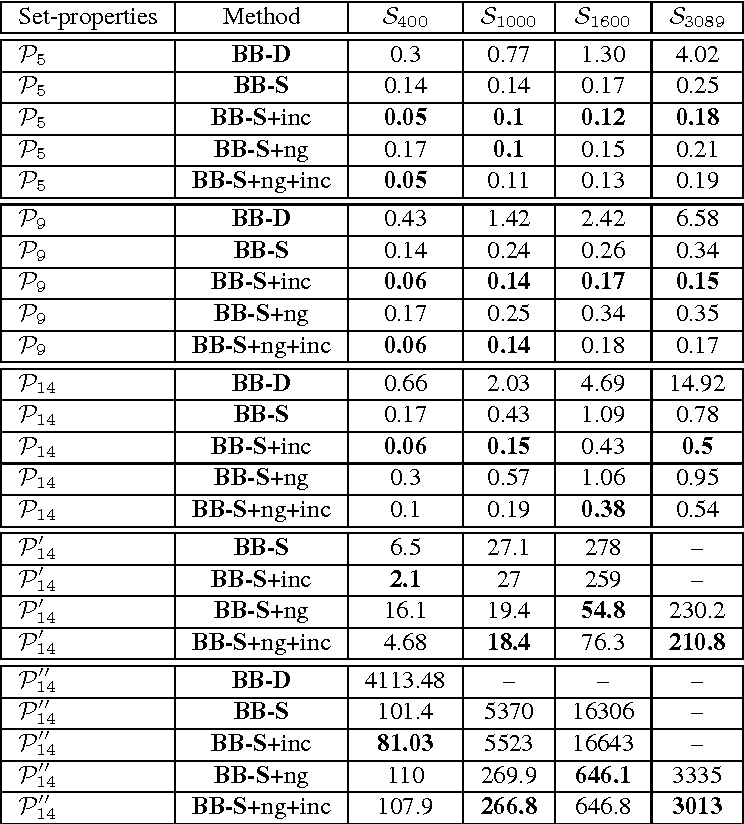Various tasks in decision making and decision support systems require selecting a preferred subset of a given set of items. Here we focus on problems where the individual items are described using a set of characterizing attributes, and a generic preference specification is required, that is, a specification that can work with an arbitrary set of items. For example, preferences over the content of an online newspaper should have this form: At each viewing, the newspaper contains a subset of the set of articles currently available. Our preference specification over this subset should be provided offline, but we should be able to use it to select a subset of any currently available set of articles, e.g., based on their tags. We present a general approach for lifting formalisms for specifying preferences over objects with multiple attributes into ones that specify preferences over subsets of such objects. We also show how we can compute an optimal subset given such a specification in a relatively efficient manner. We provide an empirical evaluation of the approach as well as some worst-case complexity results.

* Journal Of Artificial Intelligence Research, Volume 34, pages 133-164, 2009
Click to Read Paper and Get Code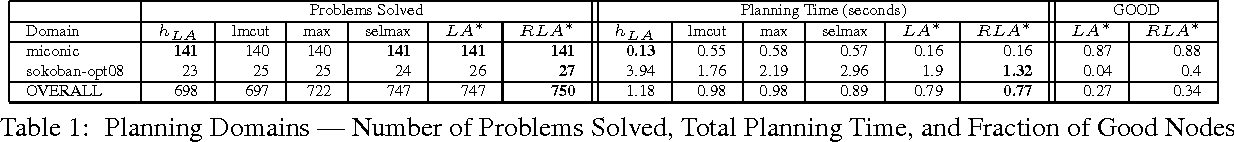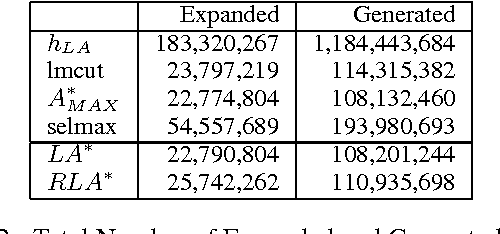The obvious way to use several admissible heuristics in A* is to take their maximum. In this paper we aim to reduce the time spent on computing heuristics. We discuss Lazy A*, a variant of A* where heuristics are evaluated lazily: only when they are essential to a decision to be made in the A* search process. We present a new rational meta-reasoning based scheme, rational lazy A*, which decides whether to compute the more expensive heuristics at all, based on a myopic value of information estimate. Both methods are examined theoretically. Empirical evaluation on several domains supports the theoretical results, and shows that lazy A* and rational lazy A* are state-of-the-art heuristic combination methods.

* 7 pages, IJCAI 2013, to appear
Click to Read Paper and Get Code